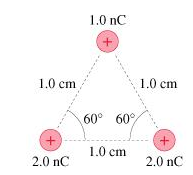# Problem: What is the magnitude of the force F on the 1.0 nC charge in the figure?

###### FREE Expert Solution

The magnitude of force:

$\overline{){\mathbf{F}}{\mathbf{=}}\frac{\mathbf{k}{\mathbf{q}}_{\mathbf{1}}{\mathbf{q}}_{\mathbf{2}}}{{\mathbf{r}}^{\mathbf{2}}}}$

Take:

q1 = 1.0 × 10-9 C, q2 = 2.0 × 10-9C, and q3 = 2.0 × 10-9C, k = 9.0 × 10kg⋅m3⋅s4⋅A2

Force of q2 on q1 :

F12 = (9.0 × 109)(1.0 × 10-9)(1.0 × 10-9)/(2.0 × 10-2)2 = 1.8 × 10-4 N

93% (215 ratings)###### Problem DetailsWhat is the magnitude of the force F on the 1.0 nC charge in the figure?